Chapter 6 Optics Ray Optics

### •Laws of reflection :-

1. The incident ray, normal and reflected ray all lie in the same plane.
2. The angle of incidence is always equal to angle of reflection.

#### •New Cartesian sign convention for spherical mirrors :-

1. All the distance measurements are done from pole of the mirror and height measurements are from the principal axis.
2. The distances measured in the direction of incident ray are positive and in opposite direction of incident ray are negative.
3. The heights measured upwards from principal axis are positive and downwards negative.

•The relation between focal length and radius of curvature of spherical mirror is f = R/2

•Mirror formula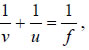where u is the object distance, v is the image distance and f is the focal length.

•Linear magnification is ratio of the size of the image to the size of the object.Magnification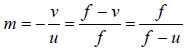If m >1 then the image is magnified.
If m<1 then the image is diminished. If m=1 then the image is of the same size of the object. If m is positive then the image is virtual and erect. If m is negative then the image is real and inverted.

•The phenomenon of bending of light at the interface of two media when it enters from one medium to another is called refraction of light.

#### •Laws of Refraction :-

1. The incident ray, normal and refracted ray all lie in the same plane.
2. The ratio of sine of angle of incidence and sine of angle of refraction is constant for a given pair of media.

#### •Snell’s law of refraction :-

The ratio of sine of angle of incidence and sine of angle of refraction is the refractive index of refracting medium with respect to the incident medium.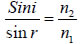•The ratio of speed of light in free space to that in medium is called absolute refractive index of that medium.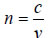•If the absolute refractive index medium 1 is greater than that of medium 2 then medium 1 is said to be optically denser medium and medium 2 is said to be optically rarer medium.

•The ratio of absolute refractive index of one medium to that of another is called relative refractive index of both the media.

###### •Principle of reversibility :-

The relative refractive index of medium 2 w.r.t. medium 1 is reciprocal to the relative refractive index of medium 1 w.r.t. medium 2.

•An object under water ( any medium ) appears to be raised due to refraction when observed inclined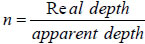and Shift in the position (apparent) of object is X = t { 1 – 1/n) where t is the actual depth of the medium.

•The Phenomenon of reflection of light completely in to the denser medium without refraction in to rarer medium is called Total Internal Reflection.

##### •Conditions for TIR :-

1. The light must propagate from optically denser medium to optically rarer medium.
2. The angle of incidence in the denser medium must be greater than critical angle of incidence for the given pair of media.

•When a ray of light travels from denser to rarer medium and if the angle of incidence is greater than critical angle, the ray of light is reflected back to the denser medium. This phenomenon is called Total internal reflection. D R n n SinC 

##### •Applications :-

Brilliance of diamond, totally reflecting prisms, Mirage, Looming, Optical Fibre.

##### Refraction through spherical surfaces :-

When light falls on a convex refracting surface, it bends and the relation between U, V and R is given by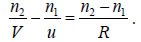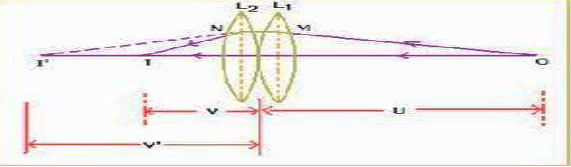•Lens maker’s formula or thin lens formula is given by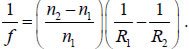•For Convex Lens R1 +ve ;R2 –ve Concave lens R1-ve; R2 +ve.

•When two lenses are kept in contact the equivalent focal length is given by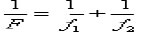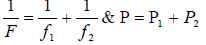•Combination of lenses help to

1)Increase the magnification of the image
2) Increase the sharpness of the final image by reducing the defects of images formed by a singe lens.
3) Make the image erect w.r.t the object
4) Increase the field of view

•The lens formula is given by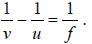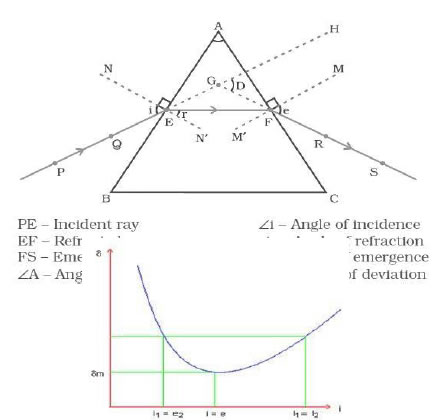•When light passes through a glass prism it undergoes refraction. The expression for refractive index is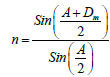As the angle of incidence increases, the angle of
deviation decreases, reaches a minimum value and then increases. This minimum value is called angle of minimum deviation “Dm”.

•The phenomenon of splitting up of polychromatic light in to its constituent colours is called Dispersion of light.

•Cause of dispersion: According to Cauchy’s formula, the refractive index (n) of a material depends upon wavelength (λ) and is given by:

•μ =a + b/λ2 + c/λ4, where a, b, c are constants.

•Scattering of light takes place when size of the particle is very small when compared to the wavelength of light. Intensity of scattered light is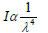•The following properties or phenomena can be explained by scattering.
1. Sky is blue.
2. Sun is reddish at the time of sunrise and sunset 3. Infra-red photography used in foggy days.
4. Orange colour of black Box
5. Yellow light used in vehicles on foggy days.
6. Red light used in signals.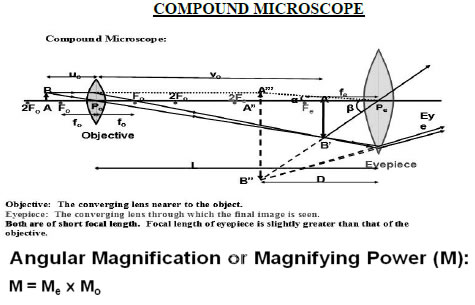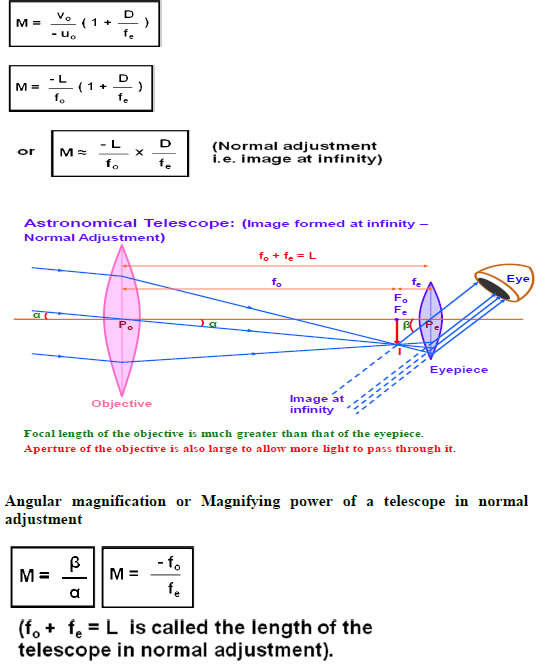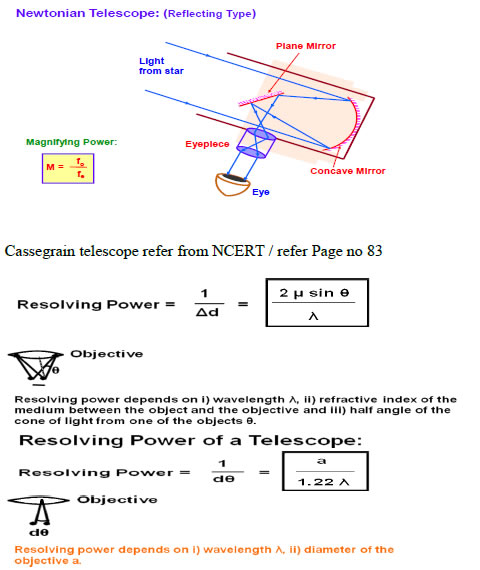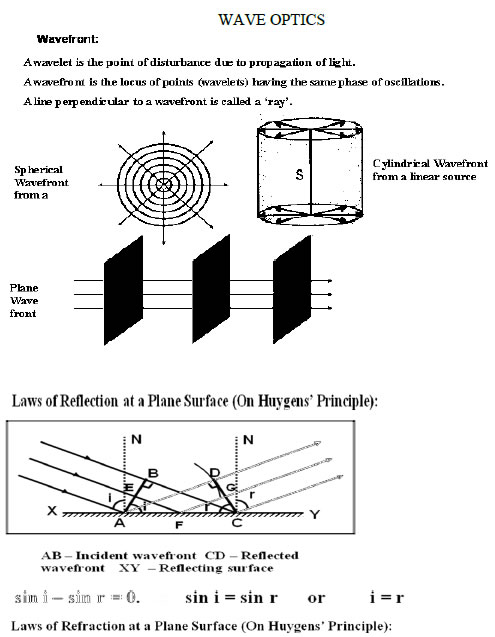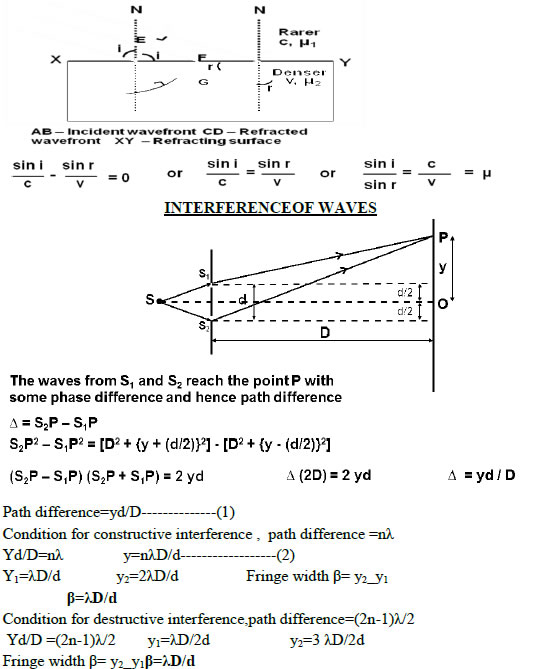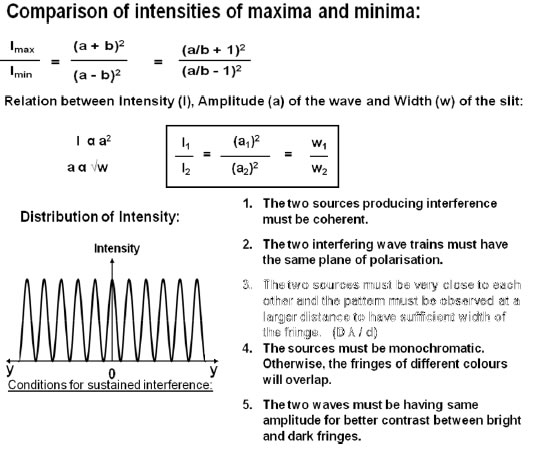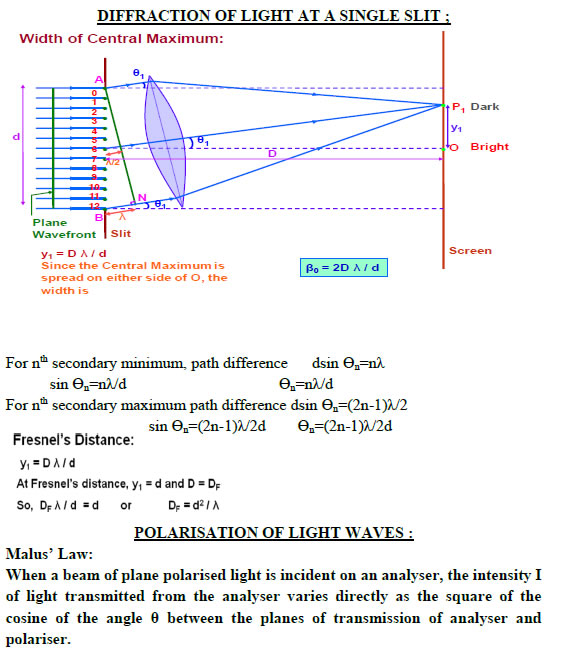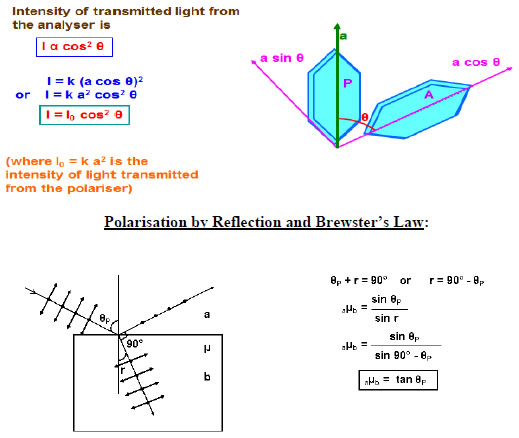##### Resolving power of microscope

Resolving power of microscope is the ability of the microscope to show as separate images of two point objects lying close to each other. Resolving power=2nsin θ/λ nsin θ is called numerical aperture of the microscope. Resolving power of telescope

##### Resolving power of telescope

is its ability to show distinctly the images of two distant objects lying close by. Resolving power =D/1.22λ D is the diameter of the objective### NCERT Books Free Pdf Download for Class 5, 6, 7, 8, 9, 10 , 11, 12 Hindi and English Medium

 Mathematics Biology Psychology Chemistry English Economics Sociology Hindi Business Studies Geography Science Political Science Statistics Physics Accountancy